# Principal Component Analysis

## Introduction to Principal Component Analysis (PCA) in Quantitative Finance

Principal Component Analysis (PCA) is a powerful statistical technique used for dimensionality reduction, allowing for the simplification of complex datasets without significantly losing the information they contain. In essence, PCA identifies patterns in data and expresses the data in such a way that it emphasizes their similarities and differences. It does so by transforming the original variables into a new set of variables called principal components, which are orthogonal to each other and capture the most significant patterns in the data.

The key strength of PCA lies in its ability to retain most of the variability present in a dataset using a reduced number of principal components. These components are ranked based on the proportion of the total variance they capture. The first principal component captures the maximum variance, the second captures the next highest, and so on.

In the field of quantitative finance, PCA plays a pivotal role:

• Risk Management: PCA can be used to understand and decompose the sources of risk in a portfolio. By analyzing the principal components, risk managers can pinpoint key factors that drive portfolio variability and then make informed decisions to mitigate those risks.
• Yield Curve Analysis: In fixed income markets, PCA is commonly used to analyze the yield curve, which represents the interest rates of bonds with different maturities. The first three principal components typically relate to the level, slope, and curvature of the yield curve, offering valuable insights for interest rate forecasting and bond portfolio structuring.
• Asset Pricing: By reducing the dimensionality of large datasets, PCA aids in identifying the dominant factors influencing asset prices. This is invaluable in building more accurate asset pricing models.
• Portfolio Optimization: For portfolios with a large number of assets, PCA can identify the primary drivers of return and risk, enabling portfolio managers to optimize asset allocation more effectively.
• Market Regime Identification: By examining how the principal components change over time, it is possible to detect shifts in market regimes, helping investors adapt their strategies accordingly.

## Mathematical Background of Principal Component Analysis (PCA)

### Linear Algebra Basics

#### Vector Spaces, Eigenvalues, and Eigenvectors

• Vector Spaces: In the context of PCA, a vector space is a collection of vectors which can be scaled and added together. This space allows us to represent multi-dimensional data as vectors, facilitating various mathematical operations.
• Eigenvalues and Eigenvectors: These are crucial concepts in linear algebra and PCA. Given a square matrix A, a vector v is an eigenvector of A if it satisfies the equation Av=λv, where λ is a scalar called the eigenvalue associated with the eigenvector v . In simpler terms, when matrix A acts upon vector v, the direction of vvdoes not change, only its magnitude may change (by a factor of λ).

#### Covariance Matrix

The covariance matrix captures the variance and correlation structure of a dataset. For n features, it’s an nxn-matrix where the element at the ith-row and jth-column represents the covariance between the its and jth feature. Diagonal elements are variances of individual features, and off-diagonal elements represent covariances between features.

### The Steps

#### Standardization

Standardization, or feature scaling, is a crucial preprocessing step for PCA. Given that PCA is sensitive to the scales of the original variables, standardization ensures that each feature contributes equally to the analysis. To ensure the PCA isn’t biased by the scale of the data, each feature should be standardized. This means each feature should have a mean μ = 0 and standard deviation σ = 1. The formula for standardizing a feature x is:

(1)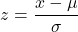Where:

•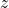​is the standardized value.
•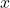​is the original value.
•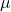is the mean of the feature.
•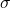is the standard deviation of the feature.

In other words, for every feature in the dataset, subtract its mean and then divide by its standard deviation.

#### Covariance Matrix Computation

Once standardized, the next step is to find the covariance matrix, which provides a measure of the relationship between pairs of features.

(2)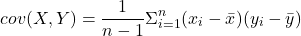Where:

• X and Y are two features.
•and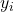are individual data points.
•andare the means of features X and Y respectively.

This matrix encapsulates the relationships between features, and its eigenvectors will point in the directions of maximum variance.

#### Eigenvalue Decomposition

At the heart of PCA is the concept of transforming the original data into a new coordinate system defined by the eigenvectors of the covariance matrix. These new coordinates are the principal components.

• Calculate the eigenvalues and eigenvectors of the covariance matrix.
• Eigenvectors represent directions of new feature space.
• Eigenvalues represent magnitude or variance in those directions.

#### Selection of Principal Components

Now, you must decide how many principal components to keep. Not all principal components are equally important.

• Rank eigenvectors by their corresponding eigenvalues in descending order.
• Examine the Scree plot: It plots the eigenvalues and helps to identify the point after which the addition of more principal components adds minimal explanatory power (the “elbow”).
• Determine the number of components, kk, such that a sufficient amount of variance (often above a threshold like 90% or 95%) is retained.

#### Transformation

After selecting the right number of principal components, transform the original data set into the new coordinate system. This is achieved by projecting the standardized data onto the selected eigenvectors.

(3)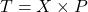Where:

•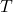is the transformed data.
•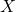is the standardized data.
•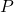is the matrix of the top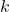eigenvectors.

The result is a dataset T with reduced dimensions, where each of the new features (principal components) is a linear combination of the original features, weighted by the corresponding eigenvector.

## Principal Component Analysis in Finance

PCA’s ability to distill vast amounts of financial data into comprehensible and actionable insights is why it’s a critical tool in the quantitative finance realm. Here are some practical examples.

### Yield Curve Analysis

The yield curve is a graphical representation that plots the yields (or interest rates) of similar-quality bonds against their maturities, ranging from the shortest to the longest. It provides an insight into how the bond market perceives future interest rate changes and economic activity. Yield curves can take various shapes—normal (upward sloping), inverted (downward sloping), or flat, depending on the relative yields of short-term and long-term bonds.

When PCA is applied to a time series of yield curves, the first few principal components often capture a significant portion of the curve’s movements:

• Level: The first principal component typically corresponds to a parallel shift in the yield curve, affecting both short-term and long-term rates almost equally. This component captures the general level of interest rates.
• Slope: The second component often represents the difference between long-term and short-term rates, effectively describing the tilt or slope of the yield curve.
• Curvature: The third component tends to capture the curvature, reflecting movements in medium-term rates relative to short-term and long-term rates.

Understanding these principal components allows fixed-income portfolio managers to make informed decisions on bond durations and maturities. By gauging how the yield curve might change in the future (shift, tilt, or twist), managers can position their portfolios optimally to maximize returns or minimize risks.

### Portfolio Management

In a diversified portfolio, it’s beneficial to have assets that are not closely correlated, ensuring that when some assets underperform, others might outperform. PCA can help identify these uncorrelated factors, revealing the underlying sources of returns in a portfolio.

By identifying the primary drivers of risk and return in a portfolio (principal components), managers can make more informed decisions on asset allocation, effectively diversifying risk. If a particular principal component is overly dominant, it might indicate a concentration of risk that needs to be addressed.

PCA can assist in the construction of factor models, which identify the primary drivers (factors) influencing asset returns. Once these factors are identified, they can be used to price assets, forecast returns, or manage risk.

### Risk Management

For portfolios with numerous assets, it can be challenging to discern where the primary risks lie. By reducing the dimensionality of the data, PCA helps risk managers isolate the main drivers of portfolio variability.

Systematic risk (or market risk) affects the entire market and cannot be diversified away. By understanding the primary components that drive portfolio variance, risk managers can monitor exposure to systematic risks and take measures, such as using derivatives or adjusting portfolio allocations, to hedge or mitigate these risks.

• Reduction of Data Dimensions
PCA is fundamentally a dimensionality reduction method. It can transform a large set of variables into a smaller one, while retaining as much of the original data’s variance as possible. By working with a reduced set of variables (principal components), computational complexity can decrease, making analyses more efficient and feasible, especially with very large datasets.
• Identification of Hidden Patterns in Data
PCA can uncover underlying structures or patterns in the data that might not be readily apparent in the original features. Unveiling these patterns can provide new insights, leading to better decision-making and forecasting.
• Helps in Understanding Correlated Features
In a dataset with multiple correlated features, PCA helps in understanding the degree and directions of those correlations. This understanding can aid in feature engineering and selection, potentially leading to more robust and simpler models.
• Improves Efficiency and Visualization
PCA can reduce the dimensions of data to two or three principal components, which can then be visualized easily. Visual representations allow for intuitive understanding, easier identification of clusters or groups, and better communication of complex data structures.